This example reanalyzes the models in Example 26.26 by using the COSAN modeling language. The correlation matrix of six variables from Kinzer and Kinzer (N=326) is used (see Guttman 1957). McDonald (1980) uses this data set to demonstrate the fitting of a factor analysis model with linear constraints on factor loadings. Two factors are assumed for the data. The factor loading matrix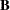is shown in the following: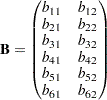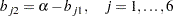The correlation structures are represented by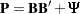where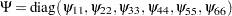represents the diagonal matrix of unique variances for the variables. Because matrix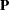is a correlation matrix, its diagonal elements are fixed constants 1. This means that the diagonal elements of the correlation structures must also satisfy the following condition: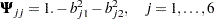To analyze the correlation structures by using PROC CALIS, you formulate a covariance structure model with such correlation structures embedded in the model. That is, you want to fit the following covariance structure model to the Kinzer data: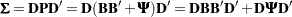where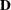is a 6 x 6 diagonal matrix that contains the population standard deviations of the observed variables.

The following statements use the COSAN modeling language to specify this covariance structure model:

```proc calis data=Kinzer nobs=326 nose;
cosan
var= var1-var6,
D(6,DIA) * B(2,GEN) + D(6,DIA) * Psi(6,DIA);
matrix B
[ ,1] = b11 b21 b31 b41 b51 b61,
[ ,2] = b12 b22 b32 b42 b52 b62;
matrix Psi
[1,1] = psi1-psi6;
matrix D
[1,1] = d1-d6;
parameters alpha (1.);

/* SAS Programming Statements to Define Dependent Parameters*/
b12  = alpha - b11;
b22  = alpha - b21;
b32  = alpha - b31;
b42  = alpha - b41;
b52  = alpha - b51;
b62  = alpha - b61;

/* 6 Constraints on Correlation structures */
psi1 = 1. - b11 * b11 - b12 * b12;
psi2 = 1. - b21 * b21 - b22 * b22;
psi3 = 1. - b31 * b31 - b32 * b32;
psi4 = 1. - b41 * b41 - b42 * b42;
psi5 = 1. - b51 * b51 - b52 * b52;
psi6 = 1. - b61 * b61 - b62 * b62;
vnames
D   = [var1-var6],
B   = [factor1 factor2],
Psi = D;
run;
```

In the PROC CALIS statement, you specify the data set by the DATA= option and the number of observations by the NOBS= option. You also use the NOSE option to suppress the printing of the standard error estimates.

In the COSAN statement, you specify the variables for the covariance structure analysis in the VAR= option. Next, you specify the covariance structure formula for the variables. When generating the covariance structure expressions for the terms, PROC CALIS examines the matrix type of the last matrix in each term to determine how the expression is generated. If the last matrix in a term is not a symmetric matrix (including diagonal or identity matrix), the transpose of the last matrix would be included in the expression. This ensures that a symmetric matrix expression is formed for the covariance structures. For example, the first term in the current covariance structure formula is D(6,DIA)*B(2,GEN). Becauseis not a symmetric matrix, the expression generated by PROC CALIS is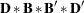However, for the second term D(6,DIA)* Psi(6,DIA), matrix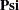is a symmetric matrix so that the expression generated by PROC CALIS is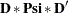Output 26.30.1 shows the covariance structure model and the model matrices. Withrepresenting the unique variance matrix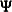, the printed covariance structure formula for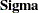is clearly what you intend to specify.

COSAN Model Structures
Sigma = D*B*B`*D` + D*Psi*D`

Summary of Model Matrices
Matrix N Row N Col Matrix Type
B 6 2 GEN: Rectangular
D 6 6 DIA: Diagonal
Psi 6 6 DIA: Diagonal

In the MATRIX statements, you specify the parameters in the model matrices. You use parameters with the b prefix to name the two columns of loadings of the factor matrix. You use free parameters psi1psi6 for the diagonal elements of thematrix, and free parameters d1d6 for the diagonal elements of thematrix. Next, you use a PARAMETERS statement to define an independent parameter alpha in the model. This parameter takes an initial value of 1.0. Using this independent parameter and six SAS programming statements, you define the loadings in the second column of matrixas functions of the loadings in the first column of the same matrix.

You use six more SAS programming statements to define the unique variance parameters psi1psi6 as dependent parameters of the factor loadings. These constraints ensure that the embedded correlation structures have diagonal elements fixed at 1.0.

Lastly, you use the VNAMES statement to label the column names of the model matrices. The column names of the diagonal matrixare the same as the observed variables. The column names of matrixare for the factor names.

As compared with the covariance structure specification (that is, the second specification) by the LINEQS model in Example 26.26, the current COSAN specification seems to be more direct and concise in specifying the parameter constraints. Because of the direct references to the matrix elements in the COSAN modeling language, you can set the required 12 constraints in a very straightforward way as the 12 SAS programming statements in the preceding specification. However, with the LINEQS model specification language in Example 26.26, you need 18 more SAS programming statements to define the correct constraints for the same covariance structure model.

Output 26.30.2 shows the fit summary table. The chi-square test statistic is 14.63 with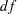=8 (= 0.067). These are the same model fitting results as using the LINEQS model specification, as shown in Output 26.26.4 of Example 26.26.

Fit Summary
Chi-Square 14.6269
Chi-Square DF 8
Pr > Chi-Square 0.0668

Output 26.30.3 shows the estimation of the loading matrix. These estimates of factor loadings are essentially the same as those obtained from the LINEQS model specification, as shown in Output 26.26.6, except that the two columns of the loading matrixare switched. The column switching is not a concern because the factor labels are arbitrary.

Output 26.30.3 Estimation of theMatrix by the COSAN Model Specification
Model Matrix B
(6 x 2 General Rectangular Matrix)
factor1 factor2
var1
 0.6318 [b11]
 0.3422 [b12]
var2
 0.6531 [b21]
 0.3210 [b22]
var3
 0.4822 [b31]
 0.4918 [b32]
var4
 0.3985 [b41]
 0.5755 [b42]
var5
 0.1971 [b51]
 0.7769 [b52]
var6
 0.3074 [b61]
 0.6666 [b62]

Output 26.30.4 shows the estimation of the scaling matrix. All these standard deviation estimates for the observed variables match those obtained from the LINEQS model specification, as shown in Output 26.26.6.

Output 26.30.4 Estimation of theMatrix by the COSAN Model Specification
Model Matrix D
(6 x 6 Diagonal Matrix)
var1 var2 var3 var4 var5 var6
var1
 1.0077 [d1]
 0
 0
 0
 0
 0
var2
 0
 0.9971 [d2]
 0
 0
 0
 0
var3
 0
 0
 0.9908 [d3]
 0
 0
 0
var4
 0
 0
 0
 0.9909 [d4]
 0
 0
var5
 0
 0
 0
 0
 0.9964 [d5]
 0
var6
 0
 0
 0
 0
 0
 1.0169 [d6]

Output 26.30.5 shows the estimation of the unique covariance matrix. All these unique variance parameter estimates match those obtained from the LINEQS model specification, as shown in Output 26.26.6.

Output 26.30.5 Estimation of theMatrix by the COSAN Model Specification
Model Matrix Psi
(6 x 6 Diagonal Matrix)
var1 var2 var3 var4 var5 var6
var1
 0.4837 [psi1]
 0
 0
 0
 0
 0
var2
 0
 0.4705 [psi2]
 0
 0
 0
 0
var3
 0
 0
 0.5256 [psi3]
 0
 0
 0
var4
 0
 0
 0
 0.5100 [psi4]
 0
 0
var5
 0
 0
 0
 0
 0.3576 [psi5]
 0
var6
 0
 0
 0
 0
 0
 0.4612 [psi6]

Finally, Output 26.30.6 shows the estimation of the independent parameter alpha. The same estimate of alpha is shown in Output 26.26.6.

Output 26.30.6 Estimation of the Independent Parameter alpha by the COSAN Model Specification